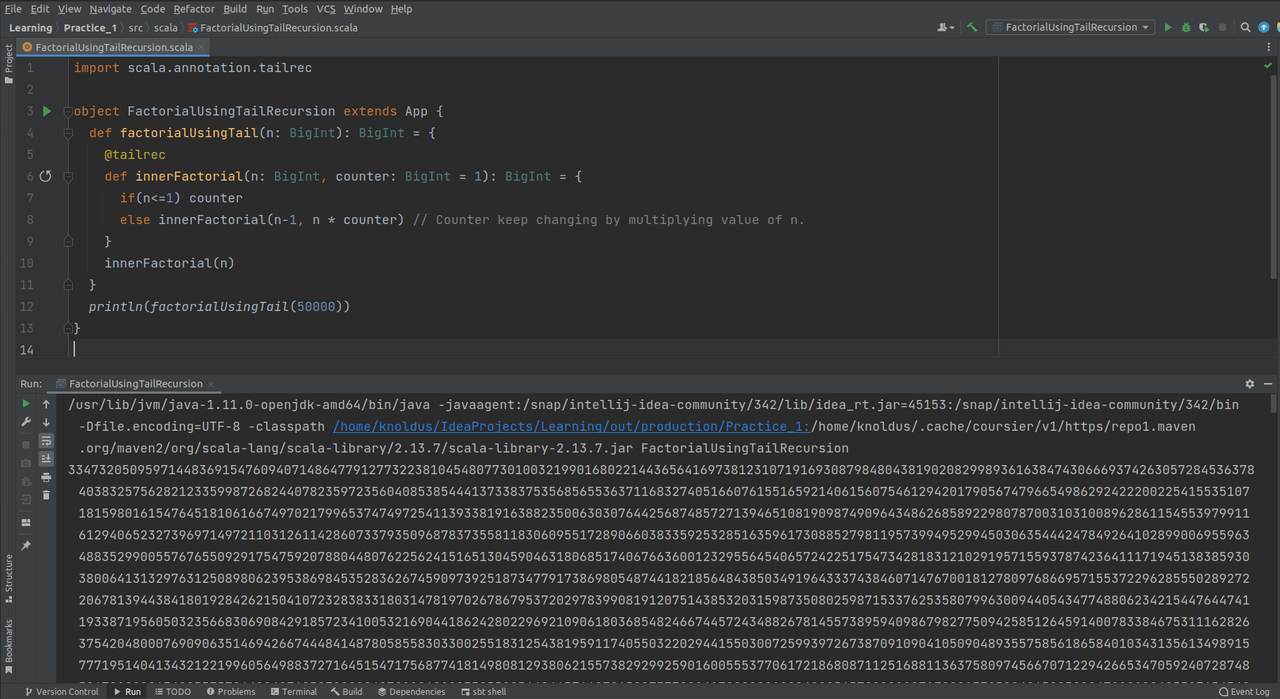## Accelerators

### Go to Overview

KDP KDSP

PremonR Studio9

#### TechHub

Akka Scala Rust Spark Functional Java Kafka Flink ML/AI DevOps Data Warehouse

## Insights# Recursion v/s Loops in ScalaReading Time: 3 minutes

Loops need mutation, As it keeps changing the value of the variable, Scala hates mutation. Why?

## What is Mutation?

A mutation is changing an object, variable and is one of the common side effects.

Now the question arises why does scala hate mutation?

Mutation might result in ambiguity, unanticipated errors, and a difficult time debugging the problem. Mutation makes it more difficult to decipher code. So the value of an object or data structure can change at any time, thus we must be cautious when reading the code.

As Scala supports Immutability, Immutability is the essential feature of scala, That’s why scala hates mutation.

Now we know why scala hates mutation, So what is the solution?
The solution is Recursion. Now the question arises

## What is Recursion?

Recursion is a widely used phenomenon in computer science used to solve complex problems by breaking them down into simpler ones. Recursion is a process by which a function calls itself directly or indirectly. The corresponding function is called a Recursive function.

### What is the base condition?

The solution to the base case is provided and the solution of the bigger problem is expressed in terms of smaller problems.

Let’s take an example to demonstrate recursion `factorial`

``````def factorial(n: Int): Int = {
if(n<=1) 1 // base condition
else n * factorial(n-1) // calling function recursively
}
println(factorial(5))``````

## Problems with Recursion

• It requires lots of memory space to hold intermediate results on the system stack.
• It is less efficient in terms of space and time complexity.
• If base class is not reachable or not defined, then it will arise the problem of stack overflow.
• Recursive functions are generally slower than non-recursive function.

## Why Stack Overflow Error Occur ?

In a recursive function, every function call will create its own stack frame in the memory and the first call of the function doesn’t return until the last call of the recursive function is resolved.

So, if the function F calls itself recursively for N times, then it would create N stack frames in the stack memory for a single execution of the function F and the compiler will keep all the N stack frames in memory till the last call of the recursive function.

## How to resolve?

To solve the stack overflow error, we have to use tail recursion. So now the question arises What is Tail Recursion?

When the last operation performed by a function is the recursive call, then it is said to be a tail-recursive function. There is no need to keep track of previous results as we use an accumulator to calculate the intermediate result. Hence, the stack memory is not much utilized.
We use @tailrec annotation to check whether a function is tail-recursive or not. But if we are using the @tailrec annotation and the function is not tail-recursive then, it will throw a compile-time error.

So let’s understand @tailrec with the help of an exampleHandled Stack Overflow Crash through Tail Recursion

## Conclusion

As we know loops cause mutation and scala adheres to the principle of immutability, Hence recursive functions are preferred over loops in scala.

So when you need loops, Use Recursion and when you need Recursion. Try using Tail Recursion.

Reference: https://docs.scala-lang.org/#### Written by Pallav Gupta

Pallav is working as Software Consultant at Knoldus Inc. He is a technology enthusiast and a keen learner with more than 1.5 years of experience. He works as a Quality Catalyst in the organization. He has a good understanding of programming languages like Java and Scala. He likes to listen to songs and playing table tennis.